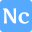## Network Calculators API

• Category: Utilities

# Public API Docs for NetworkCalc.com

NetworkCalc.com provides a public API that can be used to perform calculations related to network addresses such as IP addresses, subnet masks, and CIDR notation. In this blog post, we'll go through the available API endpoints and their usage examples in JavaScript.

## API Endpoints

Example request:

``````const address = '8.8.8.8';
fetch(url)
.then(response => response.json())
.then(data => console.log(data));
``````

### Subnet Info

Endpoint: https://api.networkcalc.com/subnet/info

Example request:

``````const subnetMask = '255.255.255.0';
fetch(url)
.then(response => response.json())
.then(data => console.log(data));
``````

### CIDR Info

This endpoint provides information about a given CIDR notation.

Endpoint: https://api.networkcalc.com/cidr/info

Example request:

``````const cidr = '192.168.0.0/24';
const url = `https://api.networkcalc.com/cidr/info?cidr=\${cidr}`;
fetch(url)
.then(response => response.json())
.then(data => console.log(data));
``````

### Subnetting

This endpoint performs subnetting on a given network.

Endpoint: https://api.networkcalc.com/subnetting

Example request:

``````const networkAddress = '192.168.0.0';
const prefixLength = '24';